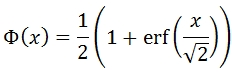# SQL Server NORMSINV function documentation

NORMSINV

Updated: 01 February 2009

Use NORMSINV to calculate the inverse of the standard normal cumulative distribution function. The equation for the cumulative distribution function is:Syntax
SELECT [wctStatistics].[wct].[NORMSINV] (
<@P, float,>)
Arguments
@P
is a probability corresponding to standard normal distribution. @P is an expression of type float or of a type that can be implicitly converted to float
Return Types
float
Remarks
·         If @P < 0 or @P > 1, NORMSINV returns an error
·         NORMSINV uses root-finding algorithms and iteration to solve for NORMSDIST(NORMINV(@Probability)) - @Probability = 0to eight decimal places

Examples

select
wct.NORMSINV(0.841344746068543)

This produces the following result

----------------------
1.00000000092742

(1 row(s) affected)

select
round(wct.NORMSDIST(wct.NORMSINV(0.841344746068543))-0.841344746068543, 9)

This produces the following result

----------------------
0

(1 row(s) affected)Copyright 2008-2020 Westclintech LLC         Privacy Policy        Terms of Service